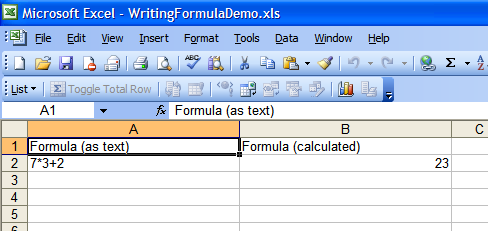Write formula into cell in XLS Excel document using Bytescout Spreadsheet SDK - ByteScout

# Write formula into cell in XLS Excel document using Bytescout Spreadsheet SDK

• Home
• /
• Articles
• /
• Write formula into cell in XLS Excel document using Bytescout Spreadsheet SDK

How to write a formula into a cell in the Excel spreadsheet generated by Bytescout Spreadsheet SDK

This source code sample demonstrates how to write formula into a cell in a new excel spreadsheet made with Bytescout Spreadsheet SDK for .NETusing System;
using System.Collections.Generic;
using System.Text;
using System.Diagnostics;

namespace HelloWorld
{
class Program
{
static void Main(string[] args)
{

// headers to indicate purpose of the column
Sheet.Cell(“A1”).Value = “Formula (as text)”;
// set A column width
Sheet.Columns.Width = 250;

Sheet.Cell(“B1”).Value = “Formula (calculated)”;
// set B column width
Sheet.Columns.Width = 250;

// write formula as text
Sheet.Cell(“A2”).Value = “7*3+2”;
// write formula as formula
Sheet.Cell(“B2”).Value = “=7*3+2”;

// save generated XLS document into new file